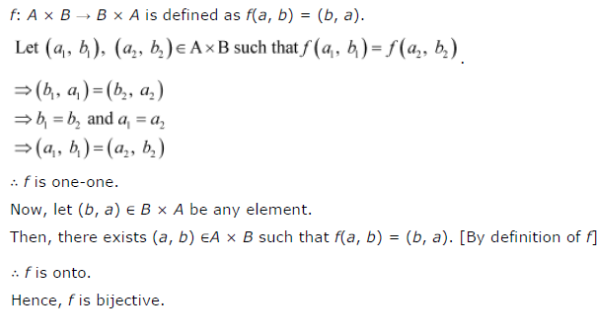# Let A and B be sets. Show that f : A × B → B × A such that f(a, b) = (b, a) is bijective function

Let A and B be sets. Show that f : A × B → B × A such that f(a, b) = (b, a) is bijective function.